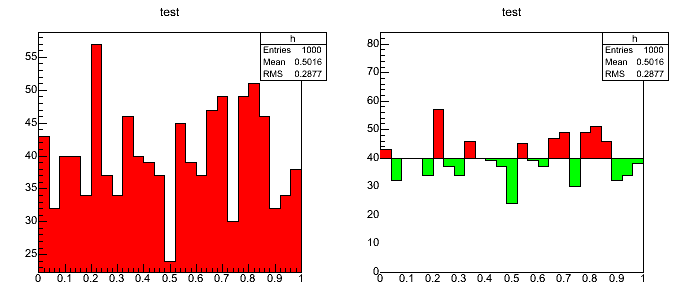# TH1: Fill deviation from zero

Dear rooters,

I want to make a histogram where the only color-filled area is the one between the histogram and a given value (say, zero) and not the area strictly below the histogram. Any suggestions?

Marcos

see a possible example below

Rene

```{ TH1F *h = new TH1F("h","test",100,-3,3); h->FillRandom("gaus",5000); h->SetFillColor(kRed); h.Draw(); TH1F *h2 = (TH1F*)h->Clone("h2"); h2->SetFillColor(kGray-4); h2->SetMaximum(40); h2->Draw("same"); }```

Hello Rene,

Thank you for your suggestion and your prompt reply. I got it to work. I’m posting an example script in case somebody else needs to produce this kind of plots.
The image shows the original histo on the left, and the output from the script on the right.

Thanks,

Marcos

```{ TH1F *h = new TH1F("h","test",25,0,1); TRandom r; TCanvas canvas("c","c",900,450); canvas.Divide(2,1); canvas.cd(1); h->Draw(); canvas.cd(2); for (int i = 0; i < 1000; i++) { h->Fill(r.Uniform()); } double Cut = 40; h->SetFillColor(kRed); TH1F *h3 = (TH1F*)h->Clone("h3"); h3->SetFillColor(kGreen); for (int n = 1; n <= h3->GetNbinsX(); n++) { h3->SetBinContent(n,Cut); } h3->Draw(); h.Draw("same"); TH1F *h2 = (TH1F*)h->Clone("h2"); h2->SetFillColor(kGray-4); h2->SetMaximum(Cut); h2->Draw("same"); }```